## Ex 5.2 Question 1.

What fraction of a clockwise revolution does the hour hand of a clock turn through, when it goes from
(a) 3 to 9
(b) 4 to 7
(c) 7 to 10
(d) 12 to 9
(e) 1 to 10
(f) 6 to 3
Solution:
(a) 3 to 9
9 – 3 = 6 ÷ 12 =$\frac { 1 }{ 2 }$ of a revolution

(b) 4 to 7
7 – 4 = 3 ÷ 12 =$\frac { 1 }{ 4 }$ of a revolution

(c) 7 to 10
10 – 7 = 3 ÷ 12 =$\frac { 1 }{ 4 }$ of a revolution

(d) 12 to 9= 0 to 9
9 – 0 = 9 ÷ 12 =$\frac { 3 }{ 4 }$ of a revolution

(e) 1 to 10
10 – 1 = 9 ÷ 12 =$\frac { 3 }{ 4 }$ of a revolution

(f) 6 to 3 = 6 to 12 and then 12 to 3
6 to 12 = 12 – 6 = 6 and 12 to 3 = 0 to 3 = 3 – 0 = 3
6 + 3 = 9 ÷ 12 =  of a revolution

## Ex 5.2 Question 2.

Where will the hand of a clock stop if it
(a) starts at 12 and makes$\frac { 1 }{ 2 }$ of a revolution, clockwise?
(b) starts at 2 and makes$\frac { 1 }{ 2 }$ of a revolution, clockwise?
(c) starts at 5 and makes$\frac { 1 }{ 2 }$ of a revolution, clockwise?
(d) starts at 5 and makes$\frac { 1 }{ 2 }$ of a revolution, clockwise?
Solution:
(a) Starting from 12 and making$\frac { 1 }{ 2 }$ of a revolution, then the clock hand stops at 6.(b) Starting from 2 and making$\frac { 1 }{ 2 }$ of a revolution, then the clock hand stops at 8.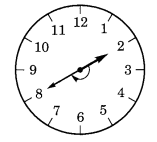(c) Starting from 5 and making$\frac { 1 }{ 2 }$ of a revolution, then the clock hand stops at 8.(d) Starting from 5 and making$\frac { 1 }{ 2 }$ of a revolution, then the clock hand stops at 2.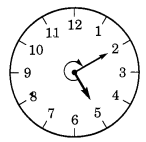## Ex 5.2 Question 3.

Which direction will you face if you start facing
(a) east and make of a revolution clockwise? z
(b ) east and make$1\frac { 1 }{ 2 }$  of a revolution clockwise? z
(c) west and make$\frac { 3 }{ 4 }$ of a revolution anticlockwise?
(d) south and make one full revolution? (Should we specify clockwise or anticlockwise for this last question? Why not?)
Solution:Taking one full revolution we will reach back to the original (starting) position. Therefore, it makes no difference whether we turn clockwise or anticlockwise.

## Ex 5.2 Question 4.

What part of a revolution have you turned through if you stand facing
(a) east and turn clockwise to face north?
(b) south and turn clockwise to face east?
(c) west and turn clockwise to face east?
Solution:
(a) If we start from east and reach north (turning clockwise)$\frac { 3 }{ 4 }$ of a revolution is required.(b) If we start from south turning clockwise to face east,$\frac { 3 }{ 4 }$ of a revolution, is required.(c) If we start from west turning clockwise to face east,$\frac { 1 }{ 2 }$ of a revolution is required.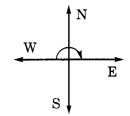## Ex 5.2 Question 5.Find the number of right angles turned through by the hour hand of a clock when it goes from
(a)3 to 6
(b) 2 to 8
(c) 5 to 11
(d) 10 to 1
(e) 12 to 9
(f) 12 to 6
Solution:
(a) 3 to 6Starting from 3 to 6, the hour hand turns through 900 or 1 right angle.

(b) 2 to 8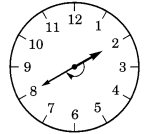Starting from 2 to 8, the hour hand turns through 1800 or 2 right angles.

(c) 5 to 11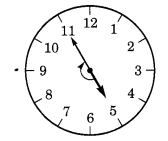Starting from 5 to 11, the hour hand turns through 1800 or 2 right angles.

(d) 10 to 1Starting from 10 to 1, the hour hand turns through 900 or 1 right angle.

(e) 12 to 9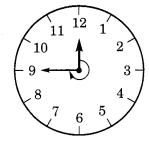Starting from 12 to 9, the hour hand turns through 2700 or  3 right angles.

(f) 12 to 6Starting from 12 to 6, the hour hand turns through 1800 or  2 right angles.

## Ex 5.2 Question 6.

How many right angles do you make if you start facing
(a) south and turn clockwise to west?
(b) north and turn anticlockwise to east?
(c) west and turn to west?
(d) south and turn to north?
Solution:## Ex 5.2 Question 7.

Where will the hour hand of a clock stop if it starts
(a) from 6 and turns through 1 right angle?
(b) from 8 and turns through 2 right angles?
(c) from 10 and turns through 3 right angles?
(d) from 7 and turns through 2 straight angles?
Solution:
(a) Starting from 6 and turning through 900 or 1 right angle, the hour hand stops at 9.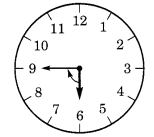(b) Starting from 8 and turning through  1800 or 2 right angles, the hour hand stops at 2.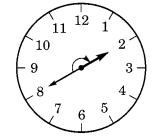(c) Starting from 10 and turning through 2700 or 3 right angles, the hour hand stops at 7.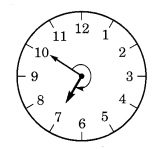(d) Starting from 7 and turning through 1800 or 2 right angles, the hour hand stops at 7.error: Content is protected !!